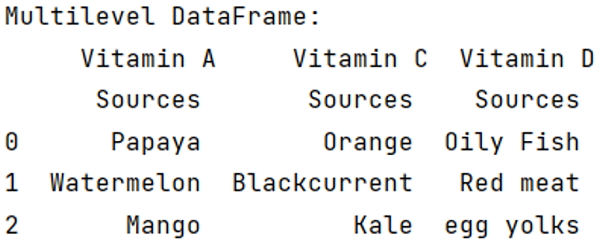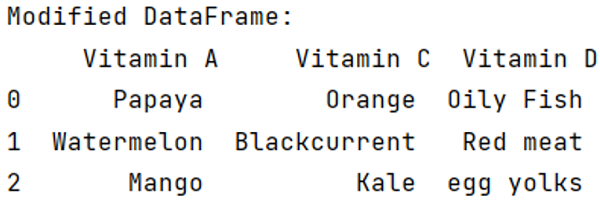# Pandas: Drop a level from a multi-level column index

Given a DataFrame, we have to drop a level from a multi-level column index. By Pranit Sharma Last updated : September 19, 2023

Columns are the different fields that contain their particular values when we create a DataFrame. We can perform certain operations on both rows & column values. In this article, we are going to learn how to drop a level from a multi-level column index.

Multilevel indexing is a type of indexing that include different levels of indexes or simply multiple indexes. The DataFrame is classified under multiple indexes and the topmost index layer is presented as level 0 of the multilevel index followed by level 1, level 2, and so on.

## Problem statement

Given a DataFrame, we have to drop a level from a multi-level column index.

## Creating a multilevel index DataFrame

To understand how to drop a level from a multilevel column index, we first need to create a multilevel index DataFrame.

Here, we are creating column wise multilevel index one below another, for this purpose, we have MultiIndex.from_tuples() method.

Note

To work with pandas, we need to import pandas package first, below is the syntax:

```import pandas as pd
```

Let us understand with the help of an example:

## Python programt to create a multilevel index DataFrame

```# Importing pandas package
import pandas as pd

# Creating multilevel index
index = pd.MultiIndex.from_tuples([('Vitamin A','Sources'),
('Vitamin C', 'Sources'),
('Vitamin D','Sources')])

# Creating a multilevel index DataFrame
# with columns = multilevel indexes
df = pd.DataFrame([['Papaya','Orange','Oily Fish'],
['Watermelon','Blackcurrent','Red meat'],
['Mango','Kale','egg yolks']], columns=index)

# Display multilevel DataFrame
print("Multilevel DataFrame:\n",df)
```

### Output## Dropping a level from a multi-level column index

For this purpose, we will use the df.columns.droplevel() method by passing the level value.

Now we will drop a level of columns from this Multiindex DataFrame

## Python program to drop a level from a multi-level column index

```# Dropping a level of column where column
# is sources i.e., level=1
df.columns = df.columns.droplevel(1)

# Display modified DataFrame
print("Modified DataFrame:\n",df)
```

### Output